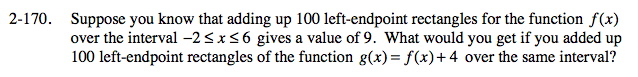### Home > PC > Chapter 2 > Lesson 2.3.8 > Problem2-170

2-170.

Suppose you know that adding up 100 left-endpoint rectangles for the function f(x) over the interval −2 ≤ x ≤ 6 gives a value of 9. What would you get if you added up 100 left-endpoint rectangles of the function g(x) = f(x) + 4 over the same interval? Homework Help ✎Use the width and height of the additional area to calculate.